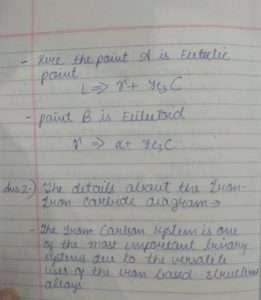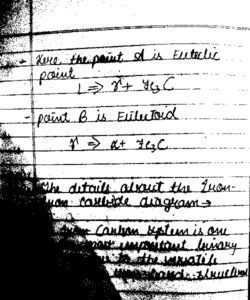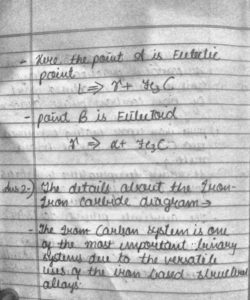# CLAHE Histogram Equalization – OpenCV

• Difficulty Level : Medium
• Last Updated : 09 Nov, 2021

In this tutorial, we are going to see how to apply Contrast Limited Adaptive Histogram Equalization (CLAHE) to equalize images. CLAHE is a variant of Adaptive histogram equalization (AHE) which takes care of over-amplification of the contrast. CLAHE operates on small regions in the image, called tiles, rather than the entire image. The neighboring tiles are then combined using bilinear interpolation to remove the artificial boundaries.
This algorithm can be applied to improve the contrast of images.

We can also apply CLAHE to color images, where usually it is applied on the luminance channel and the results after equalizing only the luminance channel of an HSV image are much better than equalizing all the channels of the BGR image.
In this tutorial, we are going to learn how to apply CLAHE and process a given input image for histogram equalization.

Parameters :
When applying CLAHE, there are two parameters to be remembered:
clipLimit – This parameter sets the threshold for contrast limiting. The default value is 40.
tileGridSize – This sets the number of tiles in the row and column. By default this is 8×8. It is used while the image is divided into tiles for applying CLAHE.

Code for above:

## Python3

 `import` `cv2` `import` `numpy as np`   `# Reading the image from the present directory` `image ``=` `cv2.imread(``"image.jpg"``)` `# Resizing the image for compatibility` `image ``=` `cv2.resize(image, (``500``, ``600``))`   `# The initial processing of the image` `# image = cv2.medianBlur(image, 3)` `image_bw ``=` `cv2.cvtColor(image, cv2.COLOR_BGR2GRAY)`   `# The declaration of CLAHE ` `# clipLimit -> Threshold for contrast limiting` `clahe ``=` `cv2.createCLAHE(clipLimit ``=` `5``)` `final_img ``=` `clahe.``apply``(image_bw) ``+` `30`   `# Ordinary thresholding the same image` `_, ordinary_img ``=` `cv2.threshold(image_bw, ``155``, ``255``, cv2.THRESH_BINARY)`   `# Showing all the three images` `cv2.imshow(``"ordinary threshold"``, ordinary_img)` `cv2.imshow(``"CLAHE image"``, final_img)`

Input image:input image

Output:Ordinary ThresholdCLAHE Applied

My Personal Notes arrow_drop_up
Recommended Articles
Page :